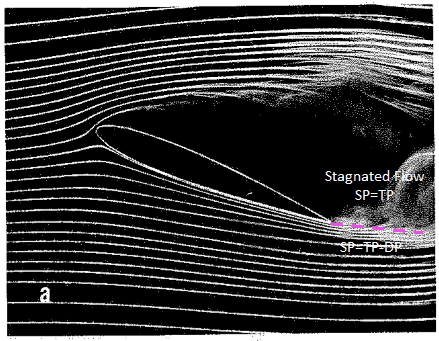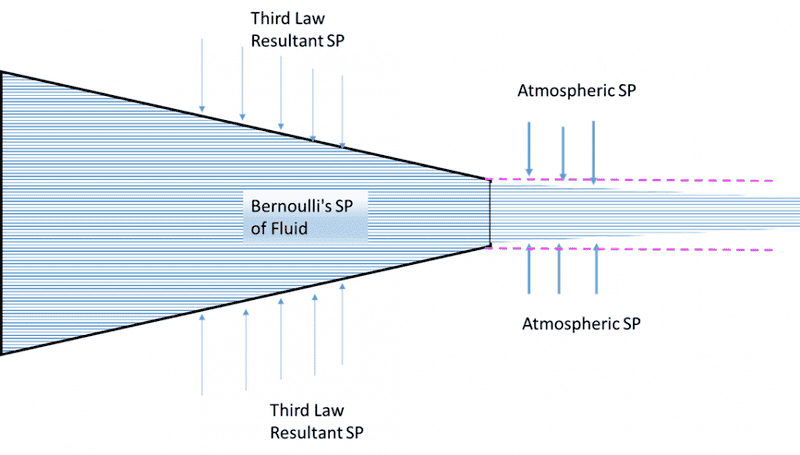Tom79Tom
Could someone explain the image we see below of a fully separated and stagnated flow over a wing
if we were to focus on where the flows rejoin on the trailing edge we see above a fully stagnated flow DP=0The static pressure here in the boundary layer above where the flows rejoin should be equal to the total pressure as there is no flow
SP=TP-0
Below we have a fast moving fluid with high DP as evidenced by tight streamlines
SP=TP-DP
If we were to draw a control surface line (pink line) parallel to the streamlines we have a Static Pressure gradient towards the higher speed lower pressure bottom flow
The image however shows the streamlines crossing the control surface into the higher pressure zone
How is this so
Is this the Kutta condition where SP at the trailing edge (where the flow rejoins) is stagnated therefore equal
If so we are seeing the diffusion (of the bottom flows) higher momentum towards the lower momentum area
If so shouldn't this be only at the stagnation point and further afield the pressure gradient would dominate ? We do see this as the lower streamlines actually become tighter past the trailing edge ?

Gold Member
What exactly are you defining as SP, TP and DP? I am just trying to make sure I am clear on your terminology.

Tom79Tom
Hi Thanks for the reply SP= static pressure TP=Total Pressure DP= Total Pressure
To explain further I am trying to understand this in comparison to what we see in a fire hose
When the velocity of the fluid in the nozzle accelerates its static pressure drops as the rigid vessel pushes back equally to the force of the fluid it when it enters the atmospheric pressure the parcel actually experiences a increase in the static pressure pushing upon it and is squeezed narrower- further speeding up the fluid parcelApplying this example I would expect to see the lower pressure/higher velocity streamlines below the wing converge past the trailing edge (being squeezed by the region of higher pressure stagnated flow above ) not spread out into the higher pressure zone as the original picture seems to indicate ?

What am I missing ?

#### Attachments

•